# Right triangle - examples - page 17

1. Chimney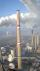Lower circumference of of the chimney is 12.57 m, top circumference is 5.655 m. The slope of the walls is 87°. Determine the height of the chimney.
2. Perimeter of triangleIn triangle ABC angle A is 60° angle B is 90° side size c is 15 cm. Calculate the triangle circumference.
3. Right triangle trigonometricsCalculate the size of the remaining sides and angles of a right triangle ABC if it is given: b = 10 cm; c = 20 cm; angle alpha = 60° and the angle beta = 30° (use the Pythagorean theorem and functions sine, cosine, tangent, cotangent)
4. ABS CNCalculate the absolute value of complex number -15-29i.
5. Hexa prismDetermine the volume of hex prism with edge base 4 cm. The body height is 28 cm.
6. Area of RT 2Calculate the area of right triangle whose legs have a length 5.8 cm and 5.8 cm.
7. Circle inscribed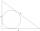Calculate the perimeter and area of a circle inscribed in a triangle measuring 3 , 4 and 5 cm.
8. CathetiThe hypotenuse of a right triangle is 41 and the sum of legs is 49. Calculate the length of its legs.
9. Is right triangleOne angle of the triangle is 36° and the remaining two are in the ratio 3:5. Determine whether triangle is a rectangular triangle.
10. Rectangular trapeziumCalculate the perimeter of a rectangular trapezium when its content area is 576 cm2 and sice a (base) is 30 cm, height 24 cm.
11. RTriangle 17The hypotenuse of a right triangle is 17 cm. If you decrease both two legs by 3 cm you will reduce the hypotenuse by 4 cm. Determine the length of this legs.
12. Right angled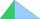From the right triangle with legs 12 cm and 20 cm we built a square with the same content as the triangle. How long will be side of the square?
13. Rotating coneCalculate the volume and the surface area of a rotating cone of base radius r = 2.3 dm and a height h = 46 mm.
14. Perimeter of circleCalculate the circumference of described circle to the triangle with sides 9,12,15 cm.
15. Inscribed circle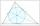Write the equation of a incircle of the triangle KLM if K [2,1], L [6,4], M [6,1].
16. The fieldThe player crossed the field diagonally and walked the length of 250 m. Calculate the length of the field, circumference if one side of field 25 meters.
17. Obtuse angle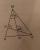The line OH is the height of the triangle DOM, line MN is the bisector of angle DMO. obtuse angle between the lines MN and OH is four times larger than the angle DMN. What size is the angle DMO? (see attached image)
18. Square circles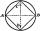Calculate the length of the described and inscribed circle to the square ABCD with a side of 5cm.
19. RhombusOne angle of a rhombus is 136° and the shorter diagonal is 8 cm long. Find the length of the longer diagonal and the side of the rhombus.
20. Hexagon rotationA regular hexagon of side 6 cm is rotated through 60° along a line passing through its longest diagonal. What is the volume of the figure thus generated?

Do you have an interesting mathematical example that you can't solve it? Enter it, and we can try to solve it.

To this e-mail address, we will reply solution; solved examples are also published here. Please enter e-mail correctly and check whether you don't have a full mailbox.

See also our right triangle calculator.